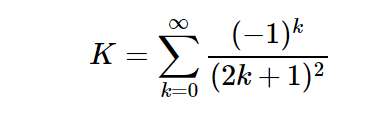# Python | sympy.S.Catalan constant

• Last Updated : 26 Jul, 2019

With the help of `sympy.S.Catalan` value, We can directly use the value of catalan constant which is K = 0.91596559 or we can say that in sympy catalan constant is a singleton value.

Formula used to calculate the catalan constant –Example #1 :
In this example we can see that by using singleton value of Catalan constant, we are able to get the value of Catalan constant.

 `# import sympy``from` `sympy ``import` `*`` ` `# Using sympy.S.Catalan value``gfg ``=` `S.Catalan.n(``10``) ``+` `S(``5``)`` ` `print``(gfg)`

Output :

5.915965594

Example #2 :

 `# import sympy``from` `sympy ``import` `*`` ` `# Using sympy.S.Catalan value``gfg ``=` `S.Catalan.n(``20``)`` ` `print``(gfg)`

Output :

0.91596559417721901505

My Personal Notes arrow_drop_up